Next: Stretching the unit circle Up: Designing filters Previous: Peaking and stop-band filter   Contents   Index

## Butterworth filters

A filter with one real pole and one real zero can be configured as a shelving filter, as a high-pass filter (putting the zero at the point) or as a low-pass filter (putting the zero at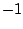). The frequency responses of these filters are quite blunt; in other words, the transition regions are wide. It is often desirable to get a sharper filter, either shelving, low- or high-pass, whose two bands are flatter and separated by a narrower transition region.

A procedure borrowed from the analog filtering world transforms real, one-pole, one-zero filters to corresponding Butterworth filters, which have narrower transition regions. This procedure is described clearly and elegantly in the last chapter of [Ste96]. The derivation uses more mathematics background than we have developed here, and we will simply present the result without deriving it.

To make a Butterworth filter out of a high-pass, low-pass, or shelving filter, suppose that either the pole or the zero is given by the expressionwhereis a parameter ranging from 1 to. If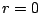this is the point, and if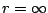it's.

Then, for reasons which will remain mysterious, we replace the point (whether pole or zero) bypoints given by:whereranges over the values:In other words,takes onequally spaced angles betweenand. The points are arranged in the complex plane as shown in Figure 8.17. They lie on a circle through the original real-valued point, which cuts the unit circle at right angles.

A good estimate for the cutoff or transition frequency defined by these circular collections of poles or zeros is simply the spot where the circle intersects the unit circle, corresponding to. This gives the point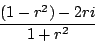which, after some algebra, gives an angular frequency equal toFigure 8.18 (part a) shows a pole-zero diagram and frequency response for a Butterworth low-pass filter with three poles and three zeros. Part (b) shows the frequency response of the low-pass filter and three other filters obtained by choosing different values of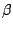(and hence) for the zeros, while leaving the poles stationary. As the zeros progress fromto, the filter, which starts as a low-pass filter, becomes a shelving filter and then a high-pass one.Next: Stretching the unit circle Up: Designing filters Previous: Peaking and stop-band filter   Contents   Index
Miller Puckette 2006-12-30